As we know, factors like strike price, price of the underlying, volatility of the underlying, time to maturity and the risk-free rate affect the Options premium. Any change in these factors will impact the Option price. Options Greeks are a group of notations that define the sensitivity of elements on the Option price.

The following are the different Options Greeks so let us learn more about them one by one.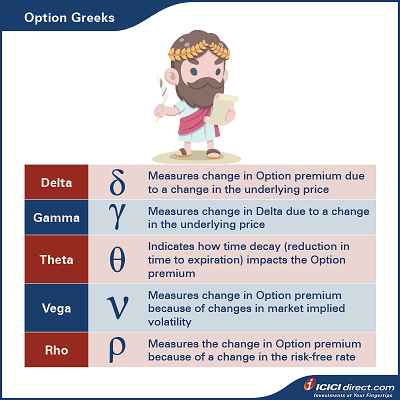## Delta

Delta calculates the extent to which the Option premium would change because of a slight change in the underlying price. For example, you might have seen that the underlying price moves up by 100 points, but the Call Option premium only moves up by 50 points. Why does it happen?

It is because of the Delta. Delta defines how much premium would change with a change in the underlying price.

Calls have a positive Delta, between 0 and 1. If a Call has a Delta of 0.60 and the stock goes up by Re. 1, in theory, the price of the Call will go up by about Rs. 0.6. If the stock goes down by Re. 1, the Call price will decrease by about Rs. 0.6. This price change will be applicable if all other pricing variables don't change.

Puts have a negative Delta, between 0 and -1. For example, if a Put has a Delta of - 0.50 and the stock goes up by Re. 1, in theory, the price of the Put will go down by Rs. 0.50.  Conversely, if the stock goes down by Re. 1, in theory, the Put price will go up by Rs. 0.50.  This price change will be applicable if all other pricing variables don't change.

Change in the price of the Option = Delta * Change in the spot price of underlying

Let's dive a bit deep into the Delta.

We know that the value of Call Options increases as the underlying stock price increase. For the deep Out of the Money (OTM) Call Options, the Option price does not change much with a change in the underlying price. It means the Delta of the OTM Call Option is close to zero. When the Call Option is deep In the Money (ITM), the Options' price almost increases in the ratio of 1:1, i.e., with every rupee increase in the underlying, Option price also increases by a rupee. It means the Delta of ITM Call Options is close to 1. It means the Call Option Delta increased from 0 to +1 for the OTM to ITM Options. The Delta of the ATM Call Option will typically be in the range of 0.4 to 0.6.

You can see the Delta and Theta value from the Option Chain available under the Option Express tab on ICICIdirect.com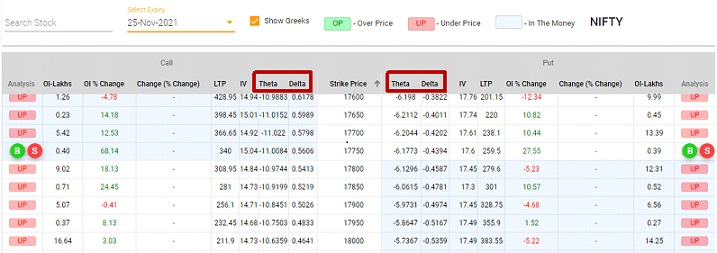*Image is for illustrative purpose only; such representations are not indicative of future results

You can also find the value of Option Greeks of a particular strike price from the Price Calculator available under the Option Express tab on ICICIdirect.com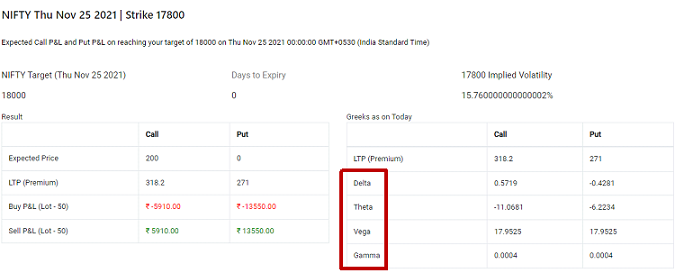*Image is for illustrative purpose only; such representations are not indicative of future resul

### But what happens in the case of a Put Option?

For deep OTM Put Options, the Option price does not change much with the change in the underlying price. It means their Delta is close to zero. For deep ITM Put Options, a change in the Options price is equivalent to a change in the underlying price, but in opposite directions. We all know that the Put Option price will fall with an increase in the underlying price. So deep ITM Put Option will have the Delta close to - 1. It means Put Option Delta remains in between 0 to - 1. The Delta of the ATM Put Option will typically stay between - 0.4 to - 0.6.

### Similar to this, what happens to Delta when Options reach near to expiry?

For OTM Call Options, Delta moves closer to zero as the Option reaches near to expiry. It is because of the assumption that the underlying stock price will not change much till expiry. For ITM Call Options, Delta moves closer to 1 as the Option reaches near to expiry. It is also because of the same assumption that the underlying price will not change much till expiry.

For OTM Put Options, Delta moves closer to zero as the Option reaches near to expiry. Similarly, for ITM Put Options, Delta moves closer to -1 as the Option reaches near to expiry. In both cases, it is because of the assumption that the underlying price will not change much till expiry.

So we conclude that the Call Option Delta remains between 0 and +1. Call Option Delta moves from 0 to +1 for the OTM to ITM Options. For Put Options, Delta remains between 0 to -1 and moves from 0 to -1 for the OTM to ITM Options.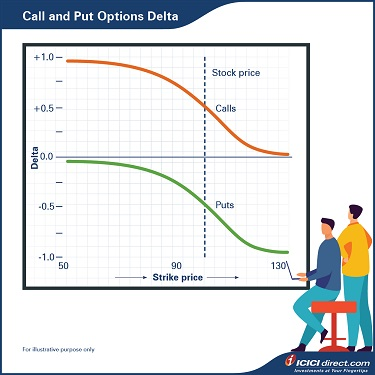## Gamma

Gamma calculates the extent to which Delta would change because of the change in the underlying price. Therefore, Gamma is considered a second-order derivative as it defines how the Delta of an Option changes.

Let's understand this with an example:

Let's say stock ABC is trading at Rs. 50. A Rs. 45 strike price Call Option trades at Rs. 6 and has a Delta of 0.80 and Gamma of 0.04. We know that for every rupee increase in the underlying, the Option price will increase equivalent to the Delta. So, If the price of the stock moves up by one rupee and reaches Rs. 51, the Call Option price will increase to 6 +0.80 = Rs. 6.80. Now at Rs. 51, Delta of the stock will change to 0.8 + 0.04 = 0.84. Gamma is responsible for a change in the Delta value.

Now let's move one step further. Gamma value is also going to change as the contract reaches near the strike price. In the above example, if the stock price falls to Rs. 49, the premium will fall to Rs. 6 - 0.8 = Rs. 5.20. Simultaneously, the Delta of the Option will also fall to 0.8 - 0.04 = 0.76. It means Delta will keep reducing as the underlying move towards the strike price.

### But does the Gamma remain the same?

No, Gamma will increase as the underlying reaches to strike price, i.e., Rs. 45 as per the example. If the Gamma remains the same, the new Delta at Rs. 45 would be 0.80 – 0.04*(50 - 45) = 0.6. But Gamma will increase and, let's say, reach 0.09 when underlying reach to Rs. 45. So typical Delta at this point will be in the range of 0.4 to 0.6. As Gamma is going to increase for every rupee fall, the actual Delta can be calculated as below with a hypothetical example: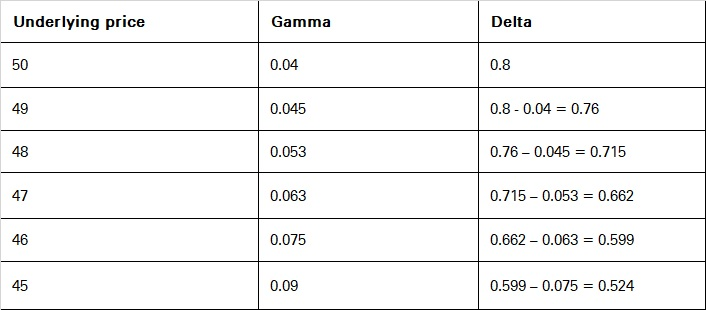So the Delta of the Option becomes 0.524 and Gamma becomes 0.09.* The above example is for illustrative purposes only; such representations are not indicative of future results.

Gamma value is maximum for At the Money (ATM) Call and Put Options. In other words, we can say that Delta is very sensitive to underlying price when the Options are At the Money. Because of the high sensitivity of the Delta, Gamma is also at maximum value for the ATM Options. Conversely, for deep ITM and OTM Options, the Gamma value approaches zero. As we know, Delta's value does not change much with a change in the underlying price for the deep ITM and OTM Options, so the Gamma value is close to zero.

### How is Gamma related to the expiry?

The change in Delta is maximum for the Options, which are At the Money and close to the expiration. So Gamma is maximum for the ATM Options which are near to expiry.

The deep ITM and OTM Options have lower Gamma. This is because as the Delta of deep ITM Call is closer to 1 and the maximum value of Delta can be 1, and there is no scope for Delta to move further if the Option becomes deeper in the money. Similarly, the deep ITM Put Options Delta is closer to -1 and the max value could be -1, so there is no further movement scope. For deep ITM Options, the change in Delta is low, so the Gamma value is also low for these Options.

For the deep OTM Call and Put Option, Delta is closer to zero, and if the Option becomes deeper OTM, Delta has no scope to change further. So Delta value will not change much, leading to a lower Gamma value.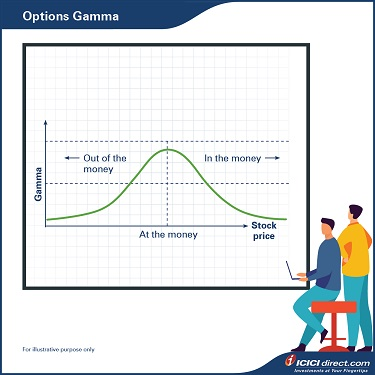## Summary

• Delta calculates the extent to which the Option premium would change because of a slight change in the underlying price.
• Call Option Delta remains between 0 and +1. Call Option Delta moves from 0 to +1 for the OTM to ITM Options.
• Put Option Delta remains between 0 to -1 and moves from 0 to -1 for the OTM to ITM Options.
• Gamma calculates the extent to which Delta would change because of the change in the underlying price.
• Gamma value is maximum for At the Money (ATM) Call and Put Options.

In the next chapter, we will discuss the Theta, Vega and Rho. It will help you understand the impact of time to maturity, interest rate and volatility on Option prices.

Disclaimer:

ICICI Securities Ltd. ( I-Sec). Registered office of I-Sec is at ICICI Securities Ltd. - ICICI Venture House, Appasaheb Marathe Marg, Prabhadevi, Mumbai - 400 025, India, Tel No : 022 - 6807 7100. I-Sec is a Member of National Stock Exchange of India Ltd (Member Code :07730), BSE Ltd (Member Code :103) and Member of Multi Commodity Exchange of India Ltd. ( Member Code : 56250) and having SEBI registration no. INZ000183631. Name of the Compliance officer (broking): Mr. Anoop Goyal, Contact number: 022-40701000, E-mail address: complianceofficer@icicisecurities.com. Investments in securities market are subject to market risks, read all the related documents carefully before investing. The contents herein above shall not be considered as an invitation or persuasion to trade or invest.  I-Sec and affiliates accept no liabilities for any loss or damage of any kind arising out of any actions taken in reliance thereon. Such representations are not indicative of future results. The securities quoted are exemplary and are not recommendatory.Option features are offered by ICICI Securities. Any complaint / dispute pertaining to the same would not be entertained by Stock Exchanges.